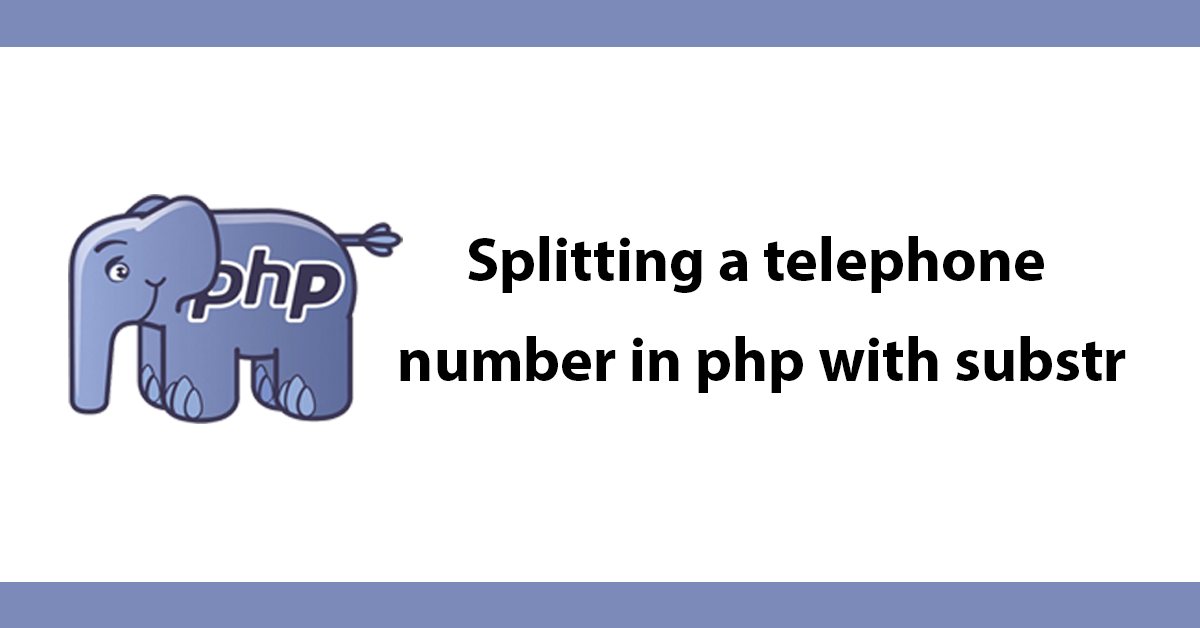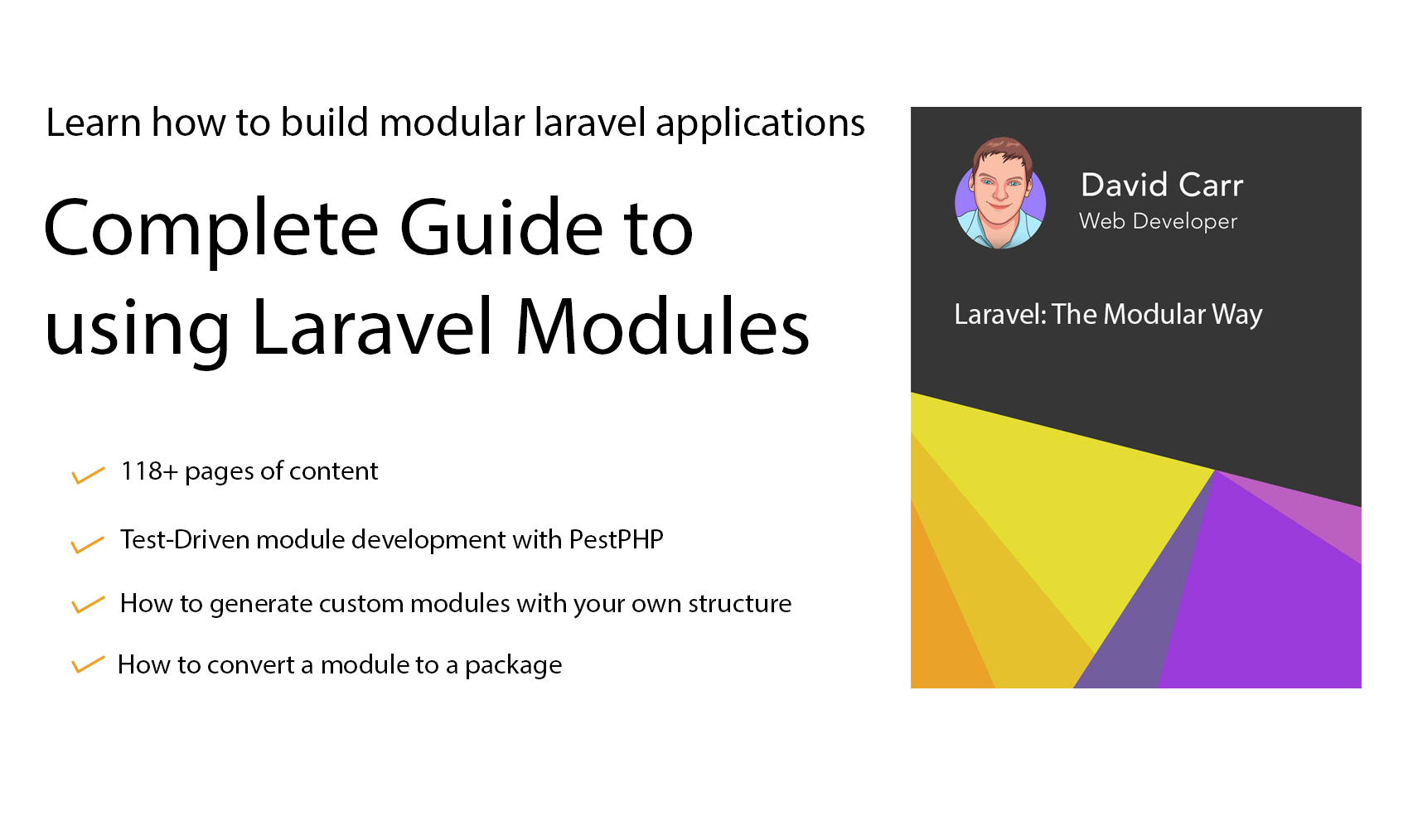# Splitting a telephone number in php with substr

I recently had a collection of numbers in the format of area code then the telephone number with no spaces such as 01482123456 I needed a space after the area code so it would read 01482 123456 this is easily achieved using PHP's substr function.

``````\$tel = '01482123456';
echo substr(\$tel, 0, 5).' '.substr(\$tel, 5, 6);``````

What the above is doing is printing the number to the page the first substr will show the area code, pass the function the number then specify where to start and how many digits to go in this case 5 then add a space then using another substr this time start at 5 digits in then and get the remainder of the number.<Help support the blog so that I can continue creating new content!